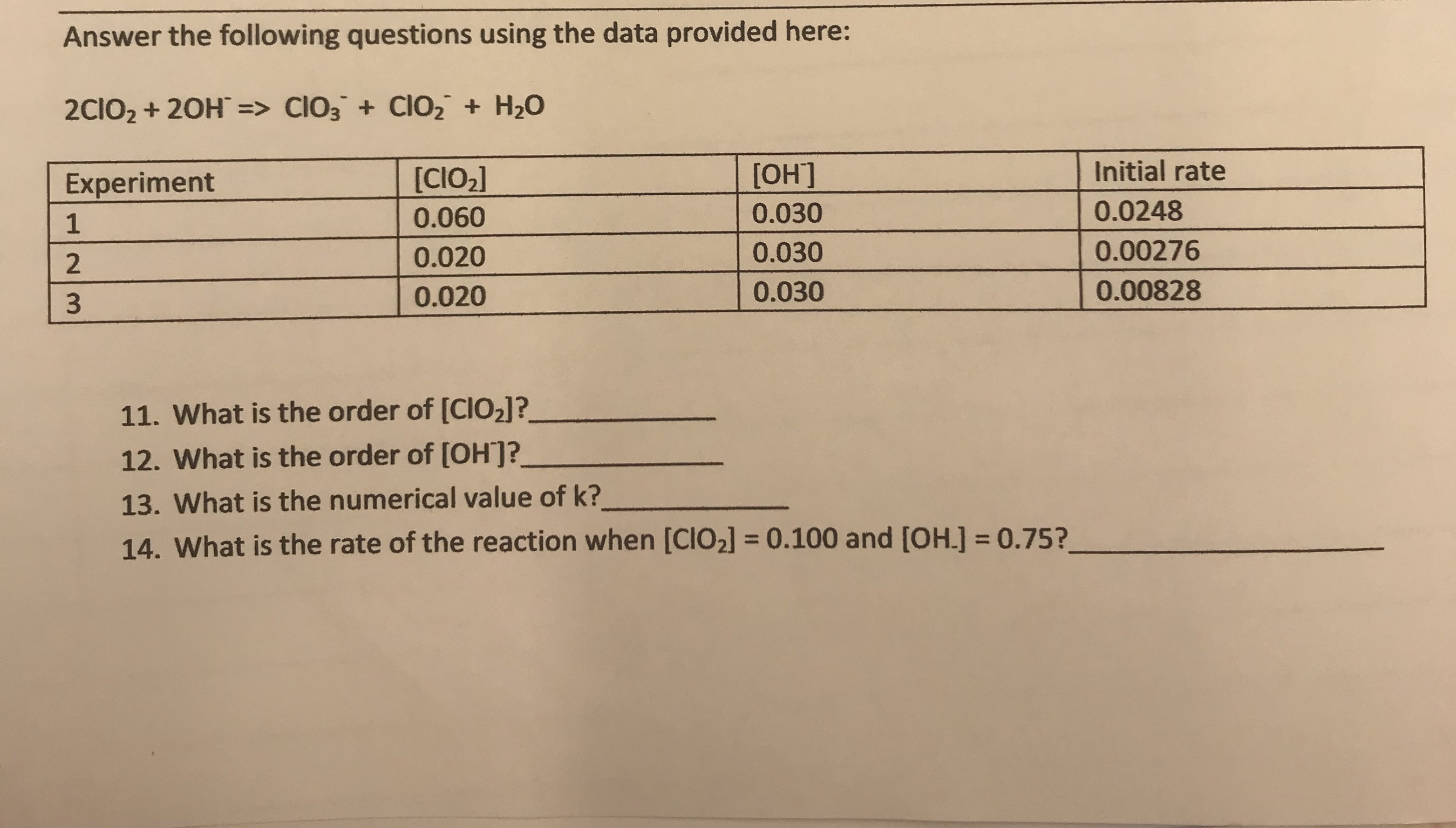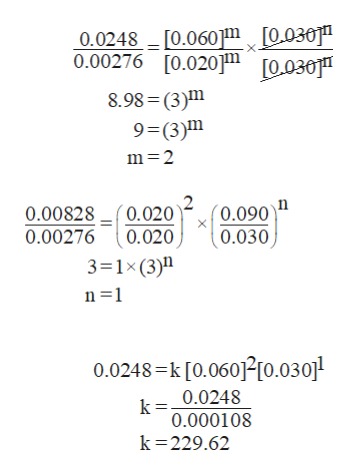Answer the following questions using the data provided here:2CIO2 20H => CIO3 Cl02 H20Initial rate[ОН][CIO2]Experiment0.02480.0300.06010.002760.0300.0200.008280.0300.02011. What is the order of [CIO2]?12. What is the order of [OH?13. What is the numerical value of k?14. What is the rate of the reaction when [CIO2] = 0.100 and [OH.] 0.75?

Question

What is the numerical value of k?help_outlineImage TranscriptioncloseAnswer the following questions using the data provided here: 2CIO2 20H => CIO3 Cl02 H20 Initial rate [ОН] [CIO2] Experiment 0.0248 0.030 0.060 1 0.00276 0.030 0.020 0.00828 0.030 0.020 11. What is the order of [CIO2]? 12. What is the order of [OH? 13. What is the numerical value of k? 14. What is the rate of the reaction when [CIO2] = 0.100 and [OH.] 0.75? fullscreen
Step 1

The given reaction in the question is

For this reaction rate of reaction is given by equation 1

Rate = K [ClO2]m[OH-]n                     ……..(1)

K is rate constant

m and n is the order of reaction with respect of  ClO2 and OH-

Order  of reaction= m + n

Step 2

From given data in question put the value from experiment 1 and 2 in equation 1

From given data in  experiment 2 and 3 in question substitutes the value in equation 1

Since from the above calculation order of reaction with respect to  [ClO2] is 2 and [OH-] is 1

So the order of reacti...help_outlineImage Transcriptionclose0.0248 _[0.060m [0.030" 0.00276 [0.020] 0030 8.98 (3)m 9=(3)m m 2 (0.090 0.030 0.00828 0.00276 0.020 0.020 3 1x (3)n n 1 0.0248 k [0.060210.030] 0.0248 0.000108 k 229.62 fullscreen

Want to see the full answer?

See Solution

Want to see this answer and more?

Our solutions are written by experts, many with advanced degrees, and available 24/7

See Solution
Tagged in

Chemical Kinetics